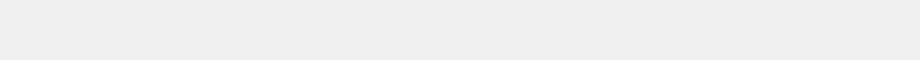Random Integers
Random integers may have a resolution, depending on the options selected, from 8 bits to 52 bits (the mantissa size of a 64-bit floating point number). Binary files are flat with no formatting which is useful if a bit stream is needed. The Hex options are scaled by the API to the maximum combinations possible for the selected resolution. When using one of the Hex options, the minimum and maximum options are ignored.
Minimum Value and Maximum Value sets the scaling range for the random numbers. These values can be any valid real number, but only the integer of the value is used. The difference between the two numbers must be at least 1.0.
How Many Numbers is the Total count of numbers. Example, 2 columns of 5 numbers, is a total of 10 numbers. Maximum count is 1,048,576 (2^20).
How Many Columns arranges the numbers into the specified number of columns.
No HTML suppresses sending HTML wrapper codes. The HTML codes enable proper display of the numbers on a web browser. HTML codes are never used when the numbers are sent as a file.
Send as Text File sends the numbers as a text file instead of sending as a web page. If the total count of numbers is greater than 16384 then the numbers are always sent as a file.
Space Separated Columns have a single space characters between numbers in a row.
Comma Separated Columns
have a comma instead of a space character separating columns.
Quoted Comma Separated columns are separated by a comma and the numbers are surrounded by the double quotes character.
JSON Array returns the numbers with the following JSON format:

{"result":[
numbers here
]}

JSON Array Quoted Numbers is the same as JSON Array but each number is surrounded by the double quotes character.
Send as Flat Binary File sends the numbers as a flattened binary file. Binary files do not have any formatting. If Integers is selected, the byte resolution will be selected by the API based on the Minimum and Maximum values.
Hex options are scaled by the API to the selected byte resolution. One Byte is scaled 0-255, Two Bytes is scaled 0-65535, and so forth. Since these options are scaled by the API, the Minimum and Maximum options are ignored.